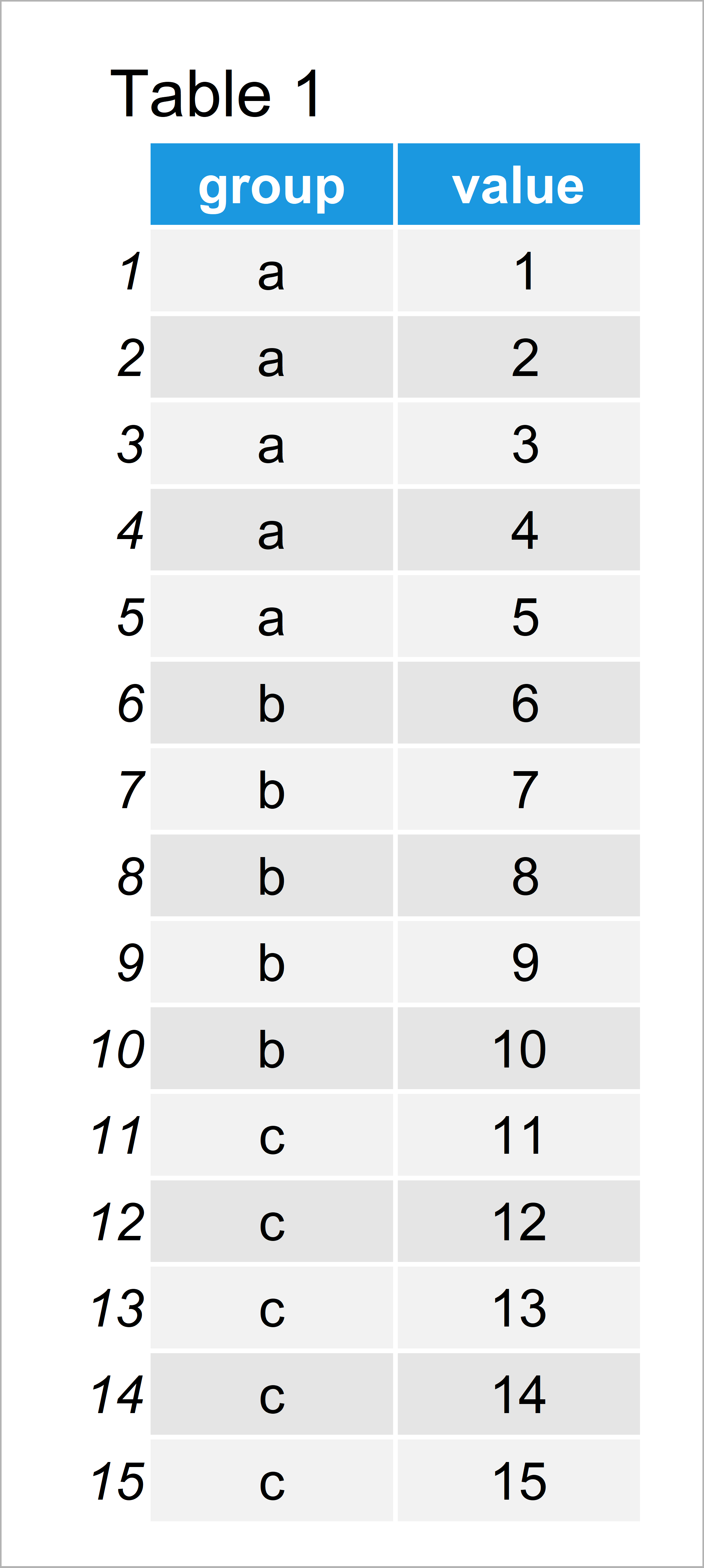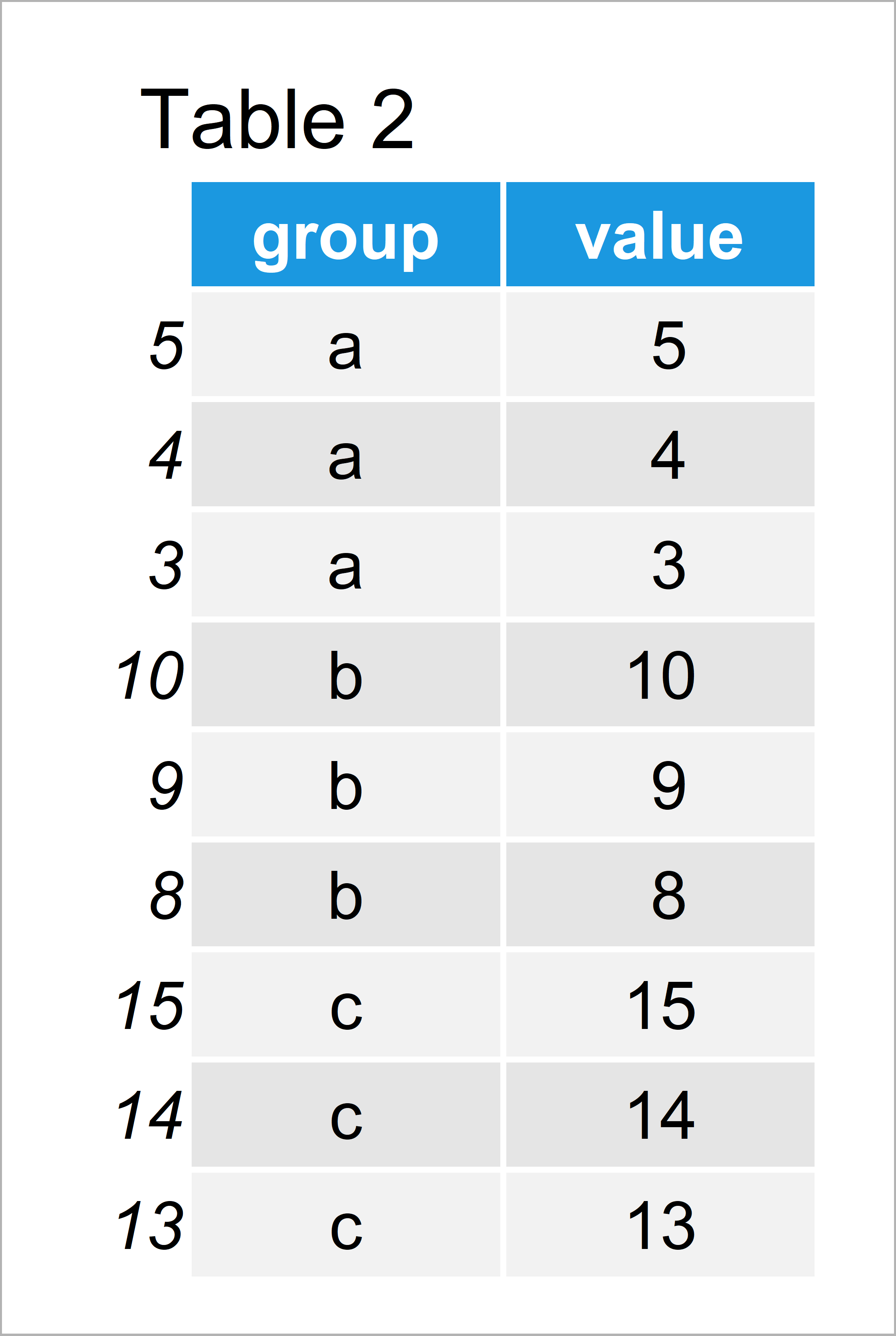# Select Top N Highest Values by Group in R (3 Examples)

This tutorial explains how to extract the N highest values within each group of a data frame column in the R programming language.

Let’s dive into it!

## Creation of Exemplifying Data

We’ll use the following data as basement for this R programming language tutorial:

```data <- data.frame(group = rep(letters[1:3], each = 5),    # Create example data
value = 1:15)
data                                                       # Print example data```As you can see based on Table 1, our example data is a data frame containing 15 rows and two columns. The variable group contains three different group indicators and the variable value contains the corresponding values.

## Example 1: Extract Top N Highest Values by Group Using Base R

In Example 1, I’ll show how to return the N highest data points of each group using the basic installation of the R programming language.

For this, we first have to sort our data based on the value column in descending order:

`data_new1 <- data[order(data\$value, decreasing = TRUE), ]  # Order data descending`

As next step, we have to apply the Reduce, rbind, and head functions as shown below:

```data_new1 <- Reduce(rbind,                                 # Top N highest values by group
by(data_new1,
data_new1["group"],
n = 3))```

The previous R code has created a new data frame object called data_new1. Let’s have a look at this data object:

`data_new1                                                  # Print updated data`The output of the previous R programming syntax is shown in Table 2 – We have created a data frame subset containing only the three cases with the highest values of each group.

## Example 2: Extract Top N Highest Values by Group Using dplyr Package

This example shows how to keep only the N observations with the highest values by group using the functions of the dplyr package.

```install.packages("dplyr")                                  # Install dplyr package

Next, we can use the arrange, desc, group_by, and slice functions to return a tibble containing only the three highest values in each group:

```data_new2 <- data %>%                                      # Top N highest values by group
arrange(desc(value)) %>%
group_by(group) %>%
slice(1:3)
data_new2                                                  # Print updated data
# # A tibble: 9 x 2
# # Groups:   group 
#   group value
#   <chr> <int>
# 1 a         5
# 2 a         4
# 3 a         3
# 4 b        10
# 5 b         9
# 6 b         8
# 7 c        15
# 8 c        14
# 9 c        13```

## Example 3: Extract Top N Highest Values by Group Using data.table Package

In this example, I’ll show how to use the data.table package to retain only the highest N values of each data frame group.

First, we need to install and load the data.table package to RStudio:

```install.packages("data.table")                             # Install data.table package

Now, we can apply the following R syntax to create a new data.table:

```data_new3 <- data[order(data\$value, decreasing = TRUE), ]  # Top N highest values by group
data_new3 <- data.table(data_new3, key = "group")
data_new3 <- data_new3[ , head(.SD, 3), by = group]
data_new3                                                  # Print updated data```After running the previous syntax the data.table containing only the three highest values in each group revealed in Table 3 has been created.

## Video & Further Resources

Have a look at the following video of my YouTube channel. In the video, I illustrate the R programming code of this article in RStudio.

Please accept YouTube cookies to play this video. By accepting you will be accessing content from YouTube, a service provided by an external third party.If you accept this notice, your choice will be saved and the page will refresh.

In addition, you could read some of the related tutorials of my website:

At this point you should know how to return the highest N values in a variable by group in the R programming language. Tell me about it in the comments below, in case you have any additional comments or questions. Furthermore, don’t forget to subscribe to my email newsletter in order to get updates on the newest posts.

Subscribe to the Statistics Globe Newsletter

•Norido Motoji
November 2, 2022 11:57 am

Hello,

Thank you so much for your article. It is really helpful for me. Now I have a problem when applying your code because my N value changes depending on the group. For example, in group a, I need 2 maximum values. In group b, I will only need 1 maximum value. What should I do in R once my value of n changes like this?

•November 14, 2022 12:47 pm